# AppleIIbot 280 char Applesoft Demos -- Part 6

## Bouncing StarsWas thinking of working on a project that had a star theme and so came up with these bouncing stars.

```1FORI=0TO140:POKE875+I,4*PEEK(2125+I)-204+(PEEK(2266+I/3)-35)/4^(I-INT(I/3)*3):NEXT
2&"/fo<HcJL54M4I:lI[N::g1nW2]L;]rJ:[4[3YGW<Ugci<]eoY:TFCEGM=.\bg2TBX4Q8LD8DdIPN;\D35E;T0X4Ui3EEW/\jU<MU7pa6iYPAm77ZZN]bH^D37V7.8rH@LU25156+357J3S+L)P80.*#L#4%^E4N8>@]5UW<M@VX%7,#50\$I%7#OK^7O_
```
Link to machine code source: stars_bot.s

## Ooze32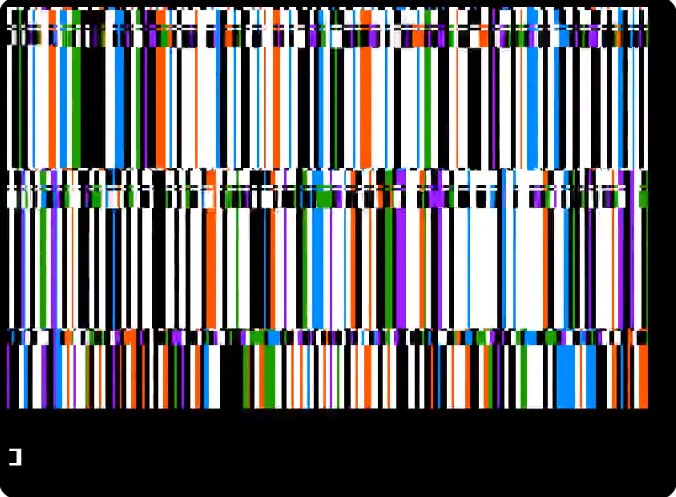This one was a total accident, and more people liked it than I expected. I forget what I was even attempting with this one. It's only 32 bytes. This broke the compression generator as it was a two digit number.

```1FORI=0TO30:POKE1013+I,4*PEEK(2125+I)-204+(PEEK(2156+I/3)-35)/4^(I-INT(I/3)*3):NEXT
2&"-hoOqTq]gNq\n:7p[3ZqW<Ugciren7l[8*<6#8%\+#
```
Link to machine code source: stars_bot.s

## Star Transition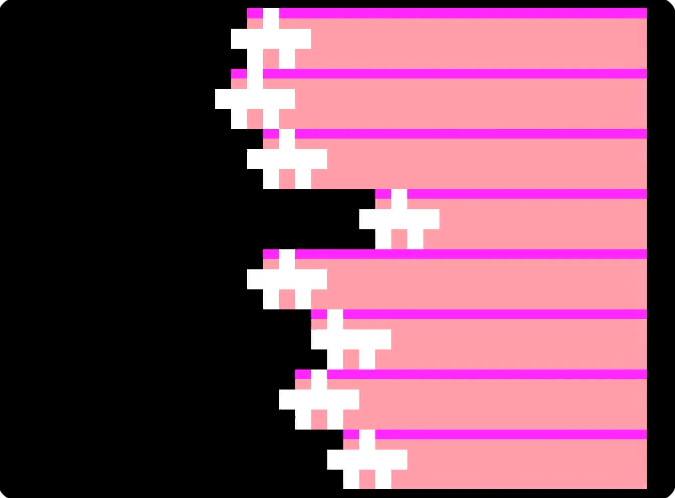This was another star themed here, just a transition.

```1FORI=0TO135:POKE880+I,4*PEEK(2125+I)-204+(PEEK(2261+I/3)-35)/4^(I-INT(I/3)*3):NEXT
2&"/@q<GcV2Tq]3]E;`q[<Y9dj3I2ES77U8rf2m2M3ES?&ZA=]3W=U1i]`VZ*`m'<98\=Dj3T=\m`o'X4EEW'ZrW=MU7parg)Z_JW3\j3`Z3QrM4L3e9gX]G;]rgU:/8<8;;;Gq;FO3S+@*%0&\;#3AE\$Z'\$;*JV'\\$:V%W\$#1XT]W07\$)#CP.&#&
```
Link to machine code source: stars_bot.s

## Secret Collect (BASIC)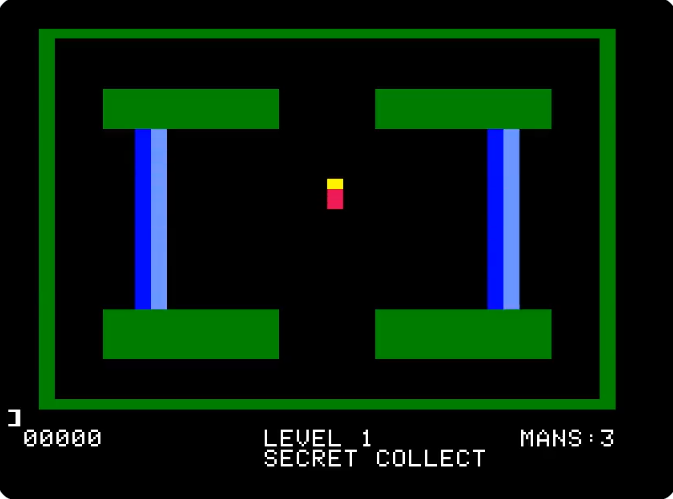Was watching some homestar runner and was thinking about maybe making an Apple ][ version, and noticed that the playfield would fit in a tweet.

In the end made an Atari 2600 version of the game instead

```0REM\$"E"G #D#F\$&A(B &A,= 16(B/(),=/>?,= 4422!4444-4411&((,=&>>,=!4433 4444')),='??,=!4422 4433 4433 4433 4433
1GR:?" 00000","LEVEL 1","MANS:3":DEFFNP(X)=PEEK(2054+I*5+X)-32
2FORI=0TO18:COLOR=FNP(0):FORY=FNP(3)TOFNP(4):HLINFNP(1),FNP(2)ATY:IFI=17THEN?,"SECRET COLLECT":I=4
3NEXTY,I
```

## Planet Hires (BASIC)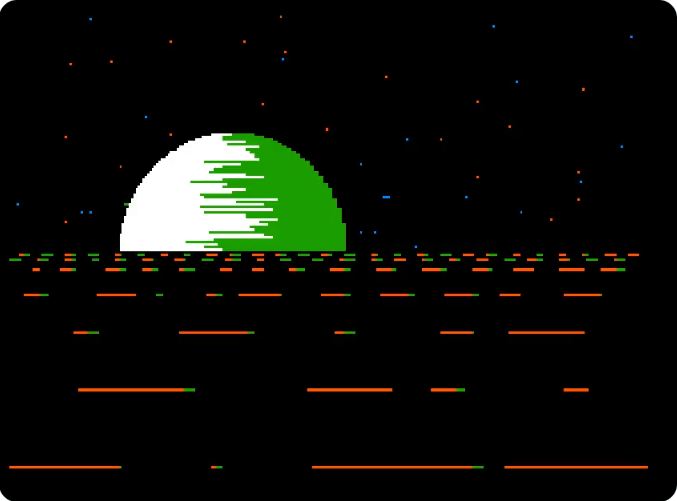The BBC Micro people found a book on planet-generating programs and were going crazy with it. It looked pretty cool so I attempted an Apple II version. In the end I mostly wrote the code from scratch, but I did re-use the clever circle drawing algorithm that was SQRT based that I hadn't seen before. I spent a bit trying to adjust the circle drawing so it finished in a timely manner but fit in 280 chars.

```0HGR2:FORR=0TO49:HPLOTRND(1)*280,RND(1)*99:NEXT
1X=X+1-(X*X/2600):Y=99-SQR(R*R-X*X):A=99+X/2-RND(1)*X:HCOLOR=3:HPLOT99-X,YTOA,Y:HCOLOR=1:HPLOTA,YTO99+X,Y
3IFX<49.9THEN1
4FORI=1TO7:X=0:T=I*I*I/4:Y=97+T
5HCOLOR=5*C:C=NOTC:Q=5+RND(1)*T:IFX+Q>279THENNEXT
6HPLOTX,YTOX+Q,Y:X=X+Q:GOTO5
```

## Planet Lores (BASIC)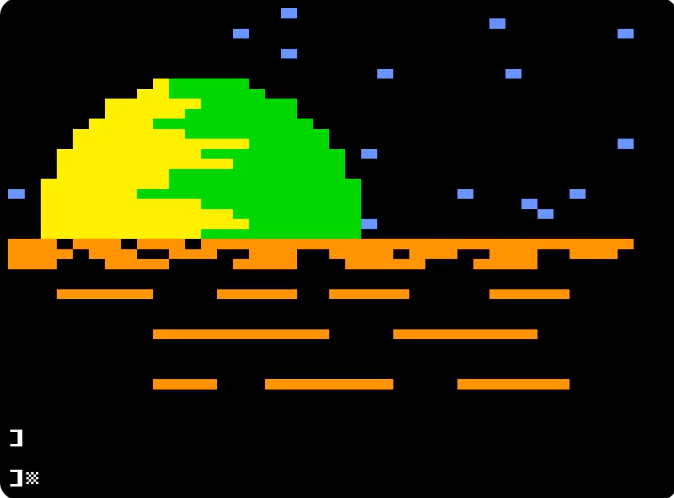I next thought maybe it would look better with more colors so tried it out in lo-res.

```0GR:COLOR=7:FORR=0TO24:PLOTRND(1)*40,RND(1)*24:NEXT
1FORX=0TO10STEP.1:Y=R-1.75*SQR(100-X*X):A=12+X/2-RND(1)*X:COLOR=13:HLIN12-X,AATY:COLOR=12:HLINA,12+XATY:NEXT
4COLOR=9:FORI=1TO7:X=0:T=I*I*I/24:Y=23+T
5C=NOTC:Q=2+RND(1)*T:IFX+Q>39THENNEXT:END
6IFCTHENHLINX,X+QATY
7X=X+Q:GOTO5
```

## Box Planet (BASIC)I made this after seeing some really neat artwork that @pixelblip1 was posting. It's a shame the bot doesn't let you pick a thumbnail time, as for this one it looked just like a blue and yellow flag.

```0REM& G 4  G55- G6O CD9;/CD88%AB;; *+;=/*+::%()== 89<>/89;;%67>> /1@E//1??%-.EE EGBF/EGAA%CDFF :=CI/:=BB%79II \$'EK/\$'DD%!#KK%!#KK
1DEFFNP(X)=PEEK(2054+I*5+X)-32:GR:POKE49234,0:FORI=0TO24:COLOR=FNP(0):FORY=FNP(3)TOFNP(4):HLINFNP(1),FNP(2)ATY:NEXTY,I:GETA
```

## Solaris1 (BASIC)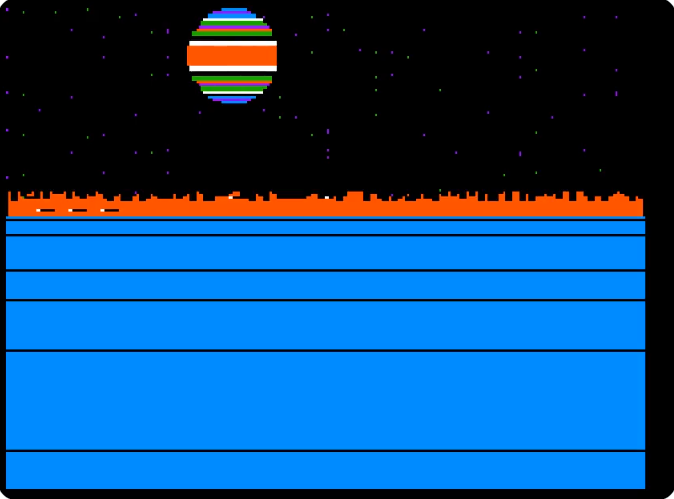Playing around with Atari stuff and wondered if I could get something Solaris-like. The clever things here are I figured out I could make the stars by blasting POKEs all over a huge chunk of memory (it's harmless to go out of bounds in this case). Also got the Y offsets for the horizon lines using a string and MID\$ which might not have been optimal.

```0HGR2:HCOLOR=5:FORX=0TO278:HPLOTX,83TOX,73+RND(1)*5:POKE16384+RND(1)*4096,1:NEXT
2A\$="*-4:DXz":HCOLOR=6:FORY=83TO191:HCOLOR=6:IFY=2*ASC(MID\$(A\$,Z+1,1))THENZ=Z+1:HCOLOR=0
6HPLOT0,YTOX,Y:NEXT
7Q=Q+0.75:Y=SQR(400-Q*Q):HCOLOR=RND(1)*7:FORT=99-QTO99+Q:HPLOTT,20-YTOT,18+Y:NEXT:GOTO7
```

## Solaris2 (BASIC)I then thought I'd try having a reversed landscape. The only clever thing here is a trick I learned from the BBC people where you can write a 4-digit number in 3, so 4E3 instead of 4096. It's not exact, but for drawing stars doesn't have to be. Made an ASCII spaceship too.

```0HGR:HCOLOR=5:FORX=0TO278:HPLOTX,83TOX,73+RND(1)*5:POKE8E3+RND(1)*4E3,1:NEXT:A\$="*-4:Dz":FORY=83TO160:HCOLOR=0:IFY=2*ASC(MID\$(A\$,Z+1))THENZ=Z+1:HCOLOR=6
3HPLOT0,YTOX,Y:NEXT
5VTAB21:?,"/\":FORQ=1TO20:Y=SQR(400-Q*Q):HCOLOR=RND(1)*8:FORT=99-QTO99+Q:HPLOTT,20-YTOT,18+Y:NEXTT,Q
6GOTO6
```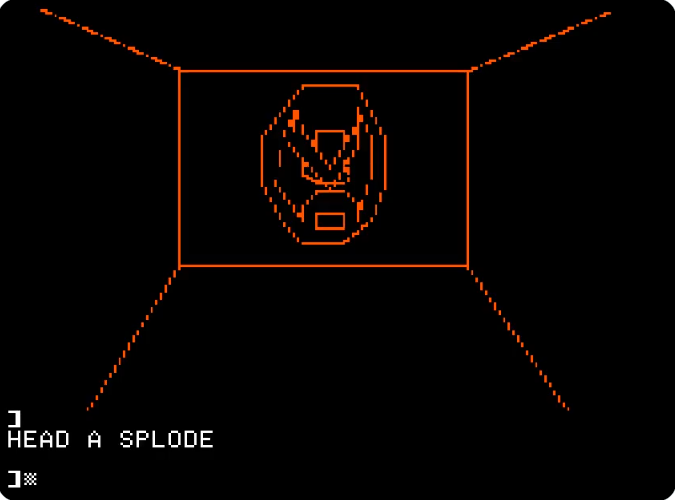Was doing more research on Secret Collect and there's another fake old game in that video, the 3-D Strongbad game. Was curious if I could do this on the Apple II and managed something almost good.

This is a new batch of code that draws hi-res coordinates based on the values in a remark. To be compact and fit in the 0..64 values you can encode in ASCII I multiply the results by 3, which means the resolution of the screen is reduced by 3. This actually made things extra tricky because orange on Apple II can only be in odd columns so really the resolution for vertical lines is 1/6. Groups of two values are read and we HPLOT TO the next point. 0 and 279 are special cased, 0 means end program and 279 means start a new point instead of drawing a connecting line segment.

```0REM~&!:):C&`~:)d)dC:C~dCz`~d)y!~L+T+X2X8V=R@L@I=F8F2L+~N<R<R>N>N<~K.I2H8L=L;N9R9T;T=V7V2T.~K.J3P9T3T.T0P6L1K.~L5L7N8R8S7R5~N3N1R1R3!
1HGR:HCOLOR=5:DEFFNP(X)=3*PEEK(2054+I+X)-99
2IFFNP(0)=279THENHPLOTFNP(1),FNP(2):I=I+3
3HPLOTTOFNP(0),FNP(1):I=I+2:IFFNP(0)GOTO2
```

## Solaris4 landscape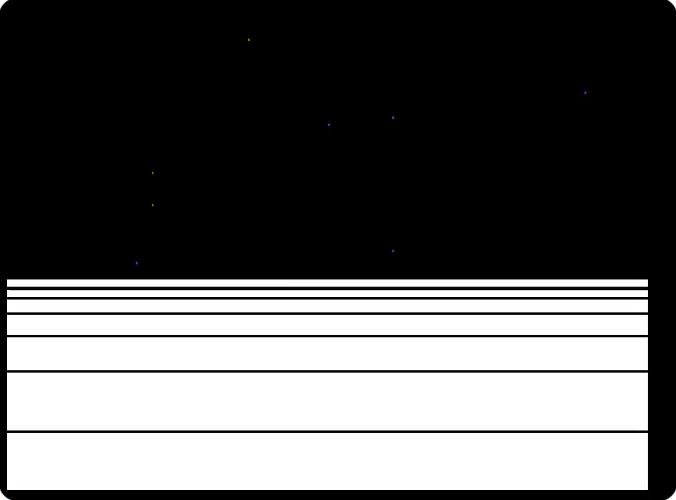Was trying to see if I could do a fast version of the solaris landscape scrolling using assembly language. Wasn't able to fit a ship in, but did something mildly clever to get some hard-to-see stars.

```1FORI=0TO140:POKE1013+I,4*PEEK(2126+I)-204+(PEEK(2267+I/3)-35)/4^(I-INT(I/3)*3):NEXT
```
Link to machine code source: solaris4.s

## Targeting Computer (BASIC)Still using the hi-res line drawing code, this time for a death star trench scene. Looks good drawn in amber.

```0REM~8)g)gX8X8)gX~82gN~8<gE~8Eg<~8Ng2~8Xg)~B3BN]N]3~I:IGVGV:~L=LDSDS=~N?NBQBQ?~D+D,N/N.O-Q.Q/[,Z+~D4D3N/N0O1Q0Q/[3Z4~M-N.~Q.R-~M1N0~Q0R1!
1HGR2:DEFFNP(X)=3*PEEK(2054+I+X)-99
2IFFNP(0)=279THENHPLOTFNP(1),FNP(2):I=I+3
3HPLOTTOFNP(0),FNP(1):I=I+2:IFFNP(0)GOTO2
```

## Elite (BASIC)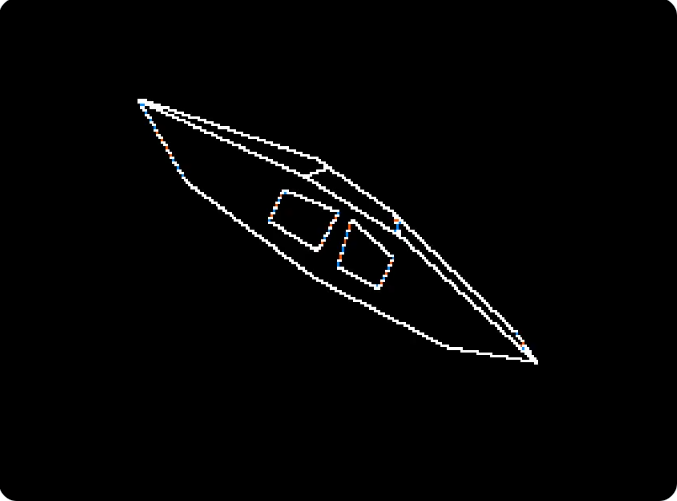The BBC Micro people are Elite-happy so I thought I'd try to get in on the action. Wanted a more interesting 3d animation but took so much code wasn't enough points left to make a smoother animation.

```1HGR2:DEFFNP(X)=3*PEEK(2197+I+X)-99
2IFI=0ORI=39THENPOKE230,32+32*P:P=NOTP:POKE49236+P,0:CALL-3086
5IFFNP(0)=6THENHPLOTFNP(1),FNP(2):I=I+3
6HPLOTTOFNP(0),FNP(1):I=(I+2)*(I<94):GOTO2"#*:B6S6l;`ALB5@):#B9B>I?I8B9#L8L?T>T:L8#4-;8NEaNnPZ?L74-N5Y<kLnP#L7O6#Z?Y<#G=I9Q<NAG=#S=YBWFQCS
```

## Mystery House (BASIC)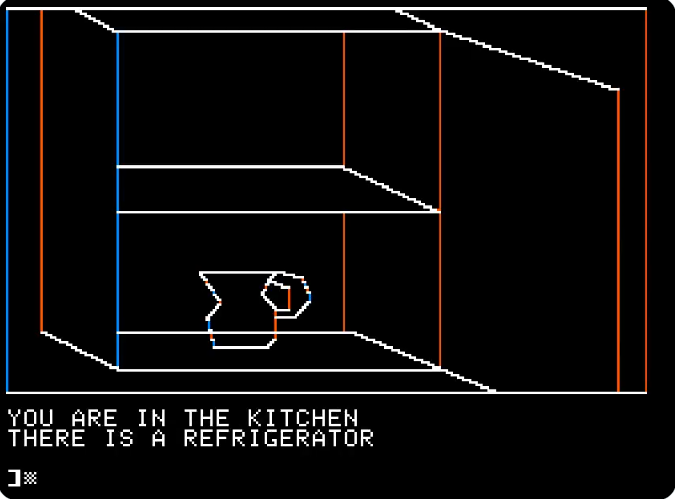A scene from the ancient Sierra Mystery House game. I tried to at Ken Williams but he ignored me.

```1HGR:DEFFNP(X)=3*PEEK(2080+I+X)-99:REM"!!~!~T!T!!"&!&L1Q1\$+!"1Q`Q`\$1\$"Y!`\$z,zT"1LSLhT"R\$R616"1<`<R6"R<RL"=DHDFGHIHMGN?N>J@H=D"HDLEMGMHKJHJHIJIJFGE
2IFFNP(0)=3THENHPLOTFNP(1),FNP(2):I=I+3
3HPLOTTOFNP(0),FNP(1):I=I+2:IFI<108GOTO2
4?"YOU ARE IN THE KITCHEN",,"THERE IS A REFRIGERATOR
```

## Spooky Office (BASIC)There was a new retro-looking game going around (I should look up the name) that had as its thumbnail an image I thought matched Apple II lo-res well.

```0REM##JJR*((*+*((.?*++(+***->*33'9*<<'=%,0(+%+1-A%49'A%=B'>#06:@)15;?#14AC&11AA&00BB#+,:A%++<<(,,?A(**EN(*/CC(49CC(99JN#=@<>%@@==+=?>>(=?<<(>@;;':::=';<990<<::
1GR:POKE49234,0:DEFFNP(X)=PEEK(2054+I*5+X)-35:FORI=0TO30:COLOR=FNP(0):FORY=FNP(3)TOFNP(4):HLINFNP(1),FNP(2)ATY:NEXTY,I
```

## Gr Xor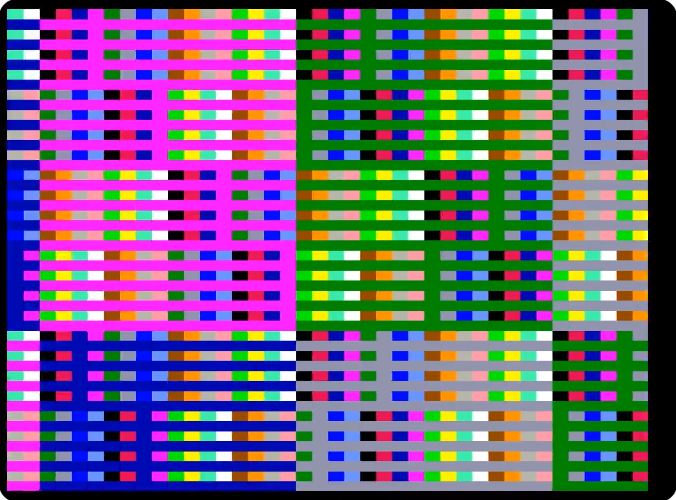```1FORI=0TO64:POKE951+I,4*PEEK(2124+I)-204+(PEEK(2189+I/3)-35)/4^(I-INT(I/3)*3):NEXT